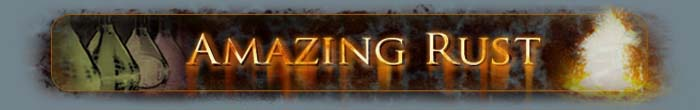Stoichiometry & Balancing Chemical Reactions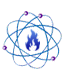Stoichiometry & Balancing Chemical Reactions

Stoichiometry refers to the quantitative relationship between reactants and products involved in a chemical reaction. One can use these stoichiometric relationships to balance a chemical reaction and determine the proper ratios between reactants.

A key concept involved in stoichiometry and balancing chemical reactions is the law of conservation of mass. In purely chemical (i.e. non-nuclear) reactions matter is only rearranged in the process, it is not created or destroyed. Furthermore, in chemical reactions, matter is only rearranged in integer-multiple numbers of whole atoms, not fractions or parts of atoms. In other words, if there are 5 of a particular type atom on the reactants side of a chemical reaction then there must also be 5 of those same atoms on the products side of the reaction even though may they be arranged (bonded) differently.

A chemical reaction may be written in the form,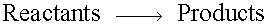Where an arrow indicates the direction of the reaction (reactants react to produce products).

Both the reactant and product sides of the above equation may contain multiple chemical compounds, so one might re-write it as,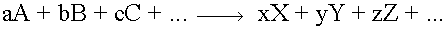where the different capital letters are intended to represent different chemicals involved in the reaction which can be, in turn, made up of various types of atoms. The lower-case letters in the above equation represent multiplicative coefficients which determine the relative amount of each of the different chemicals involved.

When balancing a chemical equation like the one shown above, the goal is to make sure for each atom on the reactants side of the equation there is a corresponding atom (of the same type / element) on the products side. This is accomplished by changing the ratios between the various chemicals involved (by changing their coefficients) in the reaction until the balance is reached.

The idea is to add coefficients in front of various chemicals, on either the reactants or products side of the chemical equation, so that the number of each type of atom is balanced. Ideally, one should balance an equation using the smallest coefficients possible. At the end, one should be able to divide the coefficients by their greatest common denominator without any effect. If the reaction is balanced properly, using the lowest possible coefficients, then the greatest common denominator will be 1 and this will have no effect, otherwise one will then obtain the lowest integer coefficients.

Examples

A few specific examples are shown below.

Consider the reaction between zinc and sulfur to produce zinc sulfide,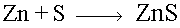One can make a table of the type, and number, of atoms on both sides of the reaction. On the reactants side there is only one, lone, atom each of both zinc and sulfur. On the products side the zinc and sulfur atoms are bonded together but there is still only one atom of each.

 Number of Atoms Reactants Type Products 1 Zn 1 1 S 1

Since there is the same number of each type of atom on both sides of the reaction it is already balanced without any modifications. One zinc and one sulfur atom react to form one formula unit of zinc sulfide.

Consider the reaction between hydrogen gas and chlorine gas to make hydrogen chloride,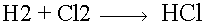Make a table showing the number of each type of atom on both sides of the reaction,

 Number of Atoms Reactants Type Products 2 H 1 2 Cl 1

There are twice as many hydrogen and chlorine atoms on the reactant side of the equation as there are on the product side. To correct this one can add a coefficient of 2 in front of the hydrogen chloride,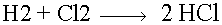Now,

 Number of Atoms Reactants Type Products 2 H 2 2 Cl 2

The reaction is now balanced. One molecule of hydrogen gas reacts with one molecule of chlorine gas to form 2 molecules of hydrogen chloride.

Now consider the reaction between hydrogen gas and oxygen gas to make water,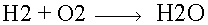Again, make a table showing the number of each type of molecule on both sides of the reaction.

 Number of Atoms Reactants Type Products 2 H 2 2 O 1

The number of oxygen atoms on the reactants side does not equal the number on the products side. To correct this one must multiply the water on the products side by placing a coefficient of 2 (meaning that there are two water molecule produced),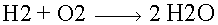Now the table of atoms becomes,

 Number of Atoms Reactants Type Products 2 H 4 2 O 2

Making this change has balanced the oxygen atoms but has un-balanced the number of hydrogen atoms. To correct this, add a coefficient of 2 to the hydrogen on the reactants side of the equation,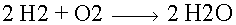The table of atoms becomes,

 Number of Atoms Reactants Type Products 4 H 4 2 O 2

Now the chemical reaction is balanced since there are 4 hydrogen atoms and 2 oxygen atoms on both sides of the equation. Two molecules of hydrogen gas react with one molecule of oxygen gas to form two molecules of water.

Last updated: 07/06/2009

This page is best viewed at a screen resolution greater than 800x600 using Internet Explorer with JavaScript enabled.

© 2005 - Amazing Rust .com International Chemical Supply    Disclaimer